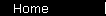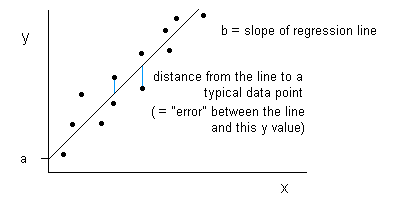### Regression

Technique of fitting a simple equation to real data points.

The most typical type of regression is linear regression (meaning you use the equation for a straight line, rather than some other type of curve), constructed using the least-squares method (the line you choose is the one that minimizes the sum of the squares of the distances between the line and the data points). It's customary to use "a" or "alpha" for the intercept of the line, and "b" or "beta" for the slope; so linear regression gives you a formula of the form:   y  =  bx + aSee r-squared, and the pages on CAPM regression and the Fama and French three factor model.

 home  |  glossary  |  calculator  |  about us  |  books